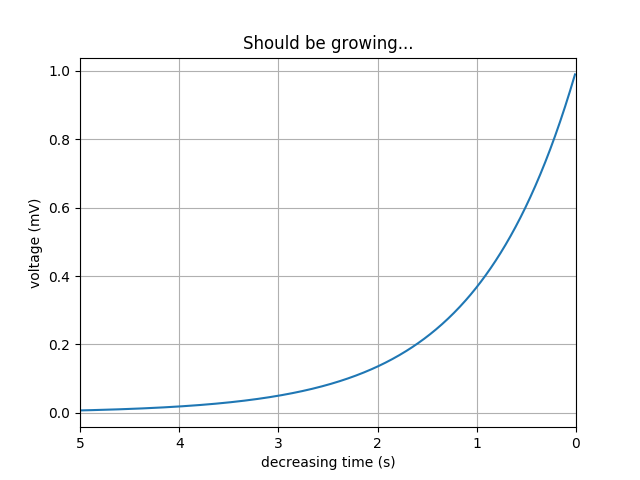Travis-CI:# Invert Axes¶

You can use decreasing axes by flipping the normal order of the axis limits```import matplotlib.pyplot as plt
import numpy as np

t = np.arange(0.01, 5.0, 0.01)
s = np.exp(-t)
plt.plot(t, s)

plt.xlim(5, 0)  # decreasing time

plt.xlabel('decreasing time (s)')
plt.ylabel('voltage (mV)')
plt.title('Should be growing...')
plt.grid(True)

plt.show()
```

Total running time of the script: ( 0 minutes 0.018 seconds)

Gallery generated by Sphinx-Gallery